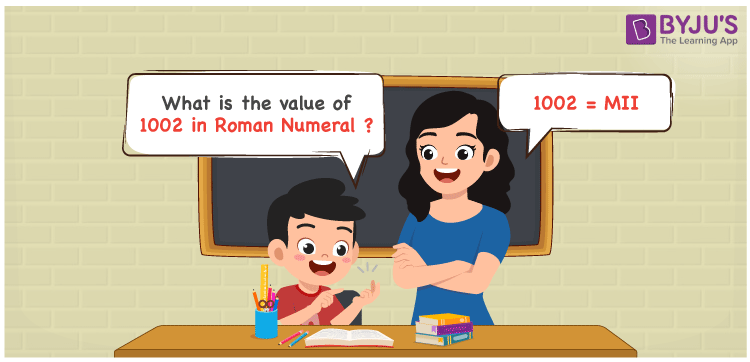# 1002 in Roman Numerals

1002 in Roman Numerals is MII. This article on the roman numerals of 1002 shows how the number is expanded based on the place value and the usage of English letters to indicate each digit. The steps to write the roman numerals are explained using an easy to understand language so that scoring good marks will be effortless. Hence, 1002 can be written as MIX in roman numerals.

 Number Roman Numeral 1002 MII## How to Write 1002 in Roman Numerals?

This section aims to improve knowledge of the numbers to roman numerals conversion among students.

1002 = 1000 + 10 + 1 + 1

1002 = M + X + I + I

1002 = MII

## Video Lesson on Roman Numerals## Frequently Asked Questions on 1002 in Roman Numerals

Q1

### Find the value of 1002 in Roman Numerals.

In the number 1002,

Thousands = 1000 = M

Ones = 2 = II

So the number = 1000 + 2 = MII

Q2

### Determine 1000 + 2.

We know that

1000 in Roman Numerals = M

2 in Roman Numerals = II

As 1000 + 2 = 1002

So 1000 + 2 is 1002 which is written as MII.

Q3

### How to write 1002 in Roman Numerals?

1002 can be written in Roman Numerals by considering the place value of each number.